In our 3-part series on "How to Assess Sensor Accuracy" this week, our CTO Paolo shares how to use R² and MAE to determine if readings from an indicative monitor are good enough to trust. If you haven't already, please read our introduction here first.

The Pearson squared correlation coefficient (R²) is calculated to determine how measurements from a device under analysis correlate with measurements of a reference instrument, or in other words, how well the device under analysis measures changes in pollutant concentration compared to the reference instrument.

The R² metric ranges from 0.0 to 1.0:

• R² close to one indicates that both the reference instrument and the device under analysis detect changes in pollutant concentration at the same time and with the same relative magnitude. If we plot the time series of the measurements from both devices, we will see the lines following the same high-low trend. If we plot the measurements from the device under analysis versus the measurements of the reference instrument in a scatter plot, the points will be arranged approximately on a line.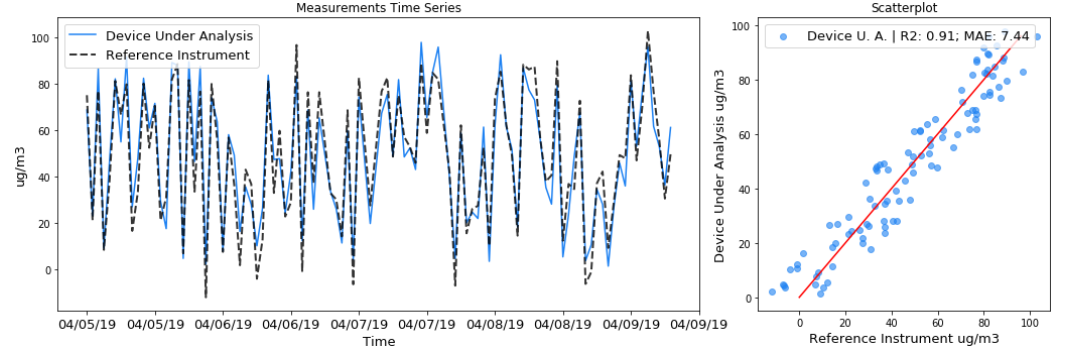Figure 1: Measurements time series and scatterplot for an imaginary device under analysis and reference instrument that are measuring PM2.5 over time. Example of a good R².

• R² close to zero indicates that when the reference instrument detects a change in pollutant concentration, the device under analysis does not, or vice versa when the reference instrument shows no change in pollutant concentration, the device under analysis measures a change. If we plot the time series of the measurements from both devices, we will see the lines following different trends with no apparent agreement. If we plot the measurements from the device under analysis versus the measurements of the reference instrument in a scatter plot, the points will be arranged in a random fashion.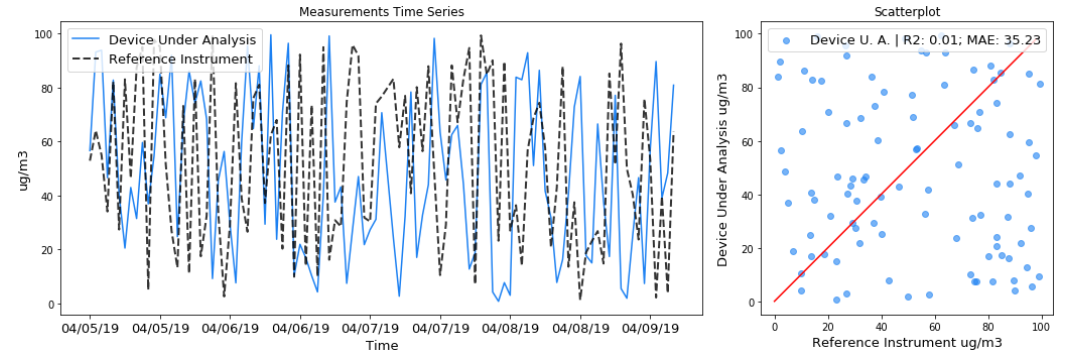Figure 2: Measurements time series and scatterplot for an imaginary device under analysis and reference instrument that are measuring PM2.5 over time. Example of a poor R².

### Limits of R²

While the R² is a common accuracy metric, it should not be used in isolation, as it does not take into account the range of pollutant concentrations that the devices are exposed to during the test.

To clarify, try to imagine an extreme case: a test where the devices are exposed to a low pollutant concentration that barely changes at all. Even if the device under analysis is very accurate, at the scale of interest its measurement time series will be a flat line, similar to the measurement time series of the reference instrument. When plotting the measurements from both devices in a scatter plot, the points will be arranged in a random cloud around a point, and the R² will be low.

In other words, since R² expresses how well the device under analysis measures changes in pollutant concentration compared to the reference instrument, this metric doesn’t work well when there is no significant change to evaluate on.

Of course, the definition of significant change depends on the phenomenon that we are trying to measure. If we are measuring PM2.5 Mass Concentration, a change of 1 or 2 𝞵g/m3 is hardly significant in terms of health effects or policy efficacy.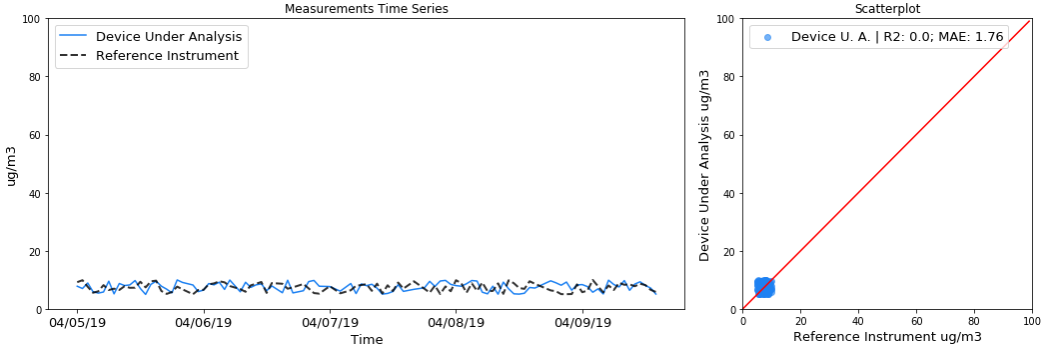Figure 3: Measurements time series and scatterplot for an imaginary device under analysis and reference instrument that are measuring PM2.5 over time. Example of a poor R² resulting from a test where the devices are exposed to a limited pollutant concentration range.

To demonstrate the sensitivity of R² on the range of pollutant concentrations, please refer to actual field data from a Clarity device and a co-located reference instrument (Figure X). The R² is 0.86 for the full dataset.

However, when a filter is applied and only the concentrations below 20 𝞵g/m3 are analyzed, the R² drops to 0.65 (Figure 2). Note that it is the same device, so it should not receive different accuracy scores depending on the range of pollutant concentrations used to test it.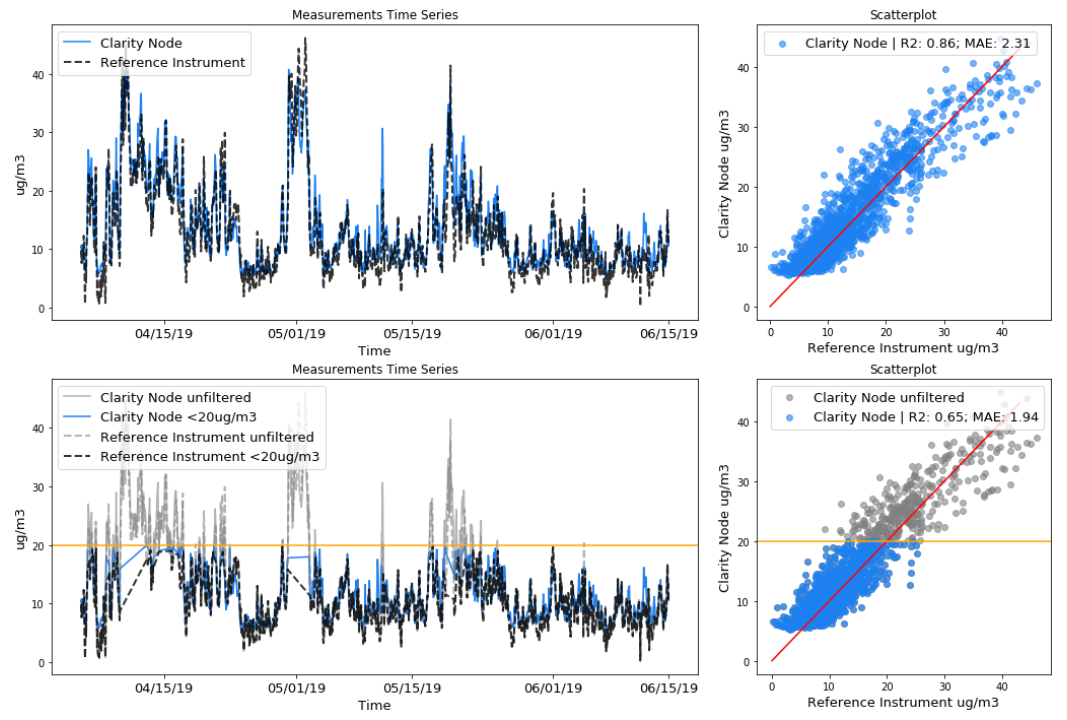Figure 4: Top: Measurements time series and scatterplot for a Clarity Node and a government reference station that are measuring PM2.5 over time. Bottom: same plots but whenever the Clarity Node reads above 20 𝞵g/m3 the data is filtered out.

A second limit of the R² metric is it only evaluates the ability of the device under analysis to detect changes in pollutant concentrations with the same relative magnitude as the reference instrument. The ability of the device under analysis to output the same absolute value as the reference instrument is not taken into account.

For example, assume that the reference instruments detect a concentration of 10 𝞵g/m3 at time t1, and of 20 𝞵g/m3 at time t2. If the device under analysis detects 1 𝞵g/m3 at time t1 and 2 𝞵g/m3 at time t2, it will receive a perfect R2 score of 1 because it was able to detect that the pollutant concentration doubled, even though the readings were off by a factor of 10. Note that this deviation can be eliminated by calibration.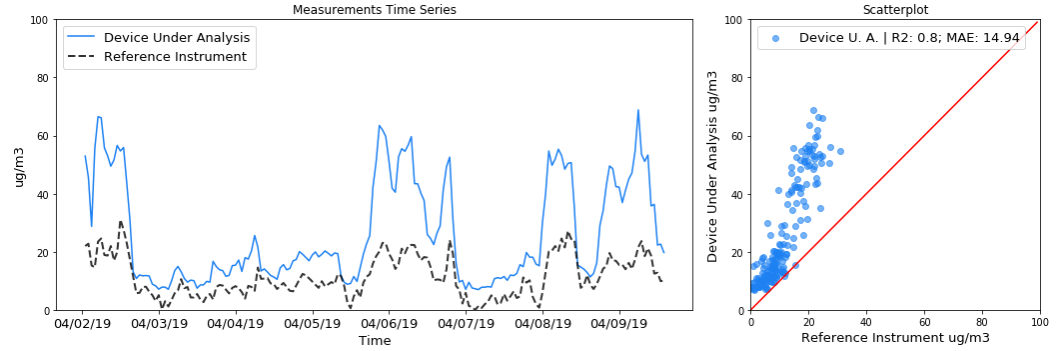Figure 5: Measurements time series and scatterplot for an imaginary device under analysis and reference instrument that are measuring PM2.5 over time. Example of good R² score and pollutant concentration overestimation.

To avoid the pitfalls of R², Clarity recommends to also calculate and report Mean Absolute Error (MAE) with R².

If you have any additional questions about R² or assessing sensor accuracy, please reach out to our team at contact@clarity.io.